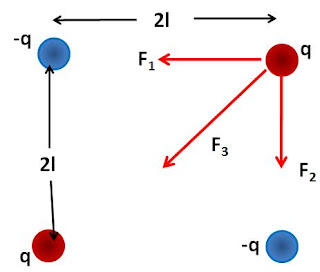## Sunday, August 22, 2010

### Irodov Problem 3.57As described in Problem 3.54, an infinite conducting surface can be treated as a mirror. In this problem there are two perpendicular semi-infinite conducting planes. So considering them as mirrors we will see two infinite perpendicular planes as shown in the figure. Similar to hall of mirrors, now the single positive charge will have 3 other image reflections as shown in the figure.The net force on the positive charge is given by the forces due to the three image charges as shown in the figure as,